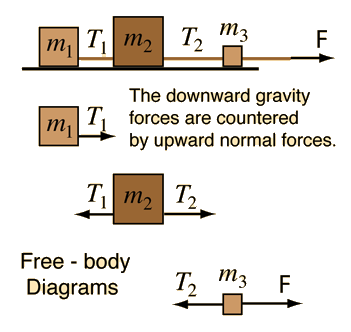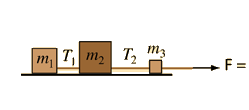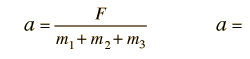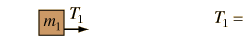# Connected MassesThe force F is the only net force acting on the system of three masses, which are constrained to accelerate together. Therefore Newton's 2nd law gives the acceleration:Once the acceleration is determined, the masses may be isolated one by one to determine the tensions T1 and T2.### Calculation

Index

Newton's laws

Standard mechanics problems

 HyperPhysics***** Mechanics R Nave
Go Back

# Connected Masses

Application of Newton's second law to connected masses. Applied force acts on masses m1 = kg, m2 =kg, m3 =kg.Nm/s²NN### Expressions

Index

Newton's laws

Standard mechanics problems

 HyperPhysics***** Mechanics R Nave
Go Back